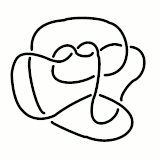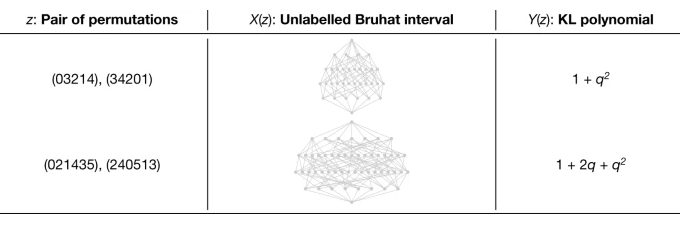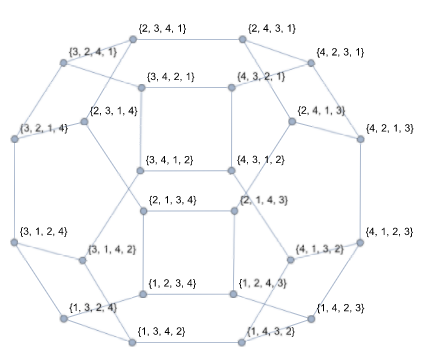# What part of AI in Math don’t you understand?!

## More about Zaka

Whether you like mathematics or not you cannot deny its effectiveness and power in making everything around us systematic with no place for chaos. But I think we have all wondered how mathematicians discover new theories and succeed in proving them. And most importantly how do these new theorems align with facts about our world that we are observing every day? I’ve always thought about Egyptians building pyramids a thousand years before Pythagoras discovered his theorem. It’s as if they knew about it somehow, and maybe they did, but couldn’t prove it.

‘I have had my results for a long time: but I do not yet know how I am to arrive at them’ ～Carl Friedrich Gauss- German Mathematician and Physicist

In mathematics, it always starts with logical reasoning with no proof or what we call ‘Conjecture’. It’s simply when someone notices a certain pattern for some cases, but this doesn’t mean it’s true for all cases; that’s when we need more data to prove it.

Here’s an example: Let’s say we have this sequence of numbers [0, 1, 4] and we want to predict the next item. To do so, we should ask ourselves how is the next number obtained? What’s the pattern here?

Is it just the square of integers like [02, 12, 22] or [0, 0x3+1, 1×3+1]? Both are actually conjectures, and we would need more data to prove which one is true.

And what’s the best way of discovering hidden patterns in the data? Of course Artificial Intelligence!

In this blog, we will answer the following questions:

• How can a supervised learning model help prove a mathematical conjecture?
• What is the Knot Theory?
• What is the Representation Theory?
• How do mathematicians use ML algorithms to prove their conjectures?

The fact of using computer software to prove mathematical conjectures is not new and actually goes back to the 1960s when German mathematician Heinrich Heesch developed a computer-assisted proof for the ‘Four color theorem’, which states that no more than four colors are needed to color any map so that adjacent regions have different colors. Since then, software in mathematics acquired a bigger role not only in theorem proving but also in identifying promising conjectures through a new algorithm called The Ramanujan Machine, named after Indian mathematician Srinivasa Ramanujan who was known for having an eye for patterns that eluded other mathematicians.

This machine was designed to generate formulas for the most famous constants such as e and 𝝅.

Actually, AI and machine learning have been used by mathematicians as essential tools for different scenarios such as accelerating calculations and detecting the existence of structure in mathematical objects. But a recent research paper published in December 2021 added another scenario where AI is used to discover new conjectures through the supervised learning framework illustrated below.

##Flowchart of the framework

Actually, AI and machine learning have been used by mathematicians as essential tools for different scenarios such as accelerating calculations and detecting the existence of structure in mathematical objects. But a recent research paper published in December 2021 added another scenario where AI is used to discover new conjectures through the supervised learning framework illustrated below.

The goal of this framework is to guide the mathematician’s intuition to verify whether a conjecture about the existence of a relationship between two mathematical objects is worth further exploration (or not), and if so, understand how they are actually related.

Ok… I know you told me from the beginning that you hate math but just be patient, it’s simpler than you think 😊

It starts with a hypothesis of an existing relationship f between two mathematical objects X(z) and Y(z) such as f(X(z)) = Y(z), which is actually done by the mathematician himself. Then according to this function, we use ML to generate more data resulting in a particular data distribution Pz which is fed to a supervised machine learning model. Trained on this pair of data [X(z);Y(z)], the supervised regression model estimates a function  and generates new Y(z) once fed X(z) only.

The benefit of using ML at this stage is that we can end up with models that can learn a broad range of possible nonlinear functions, .

If the learned function f̂ is accurate, then mathematicians can explore it through the attribution technique and formulate a candidate function, f’ which is the conjecture. This step is done by looking for the features or components of the input X(z) that contribute the most to Y(z). The suggested approach is gradient saliency, which simply calculates the derivative of the output with respect to each component of the input.

The process is iterative until the mathematician understands existing relationships or patterns, reformulates a better conjecture that fits the data, and orients his future work and research towards proving a new theorem.

Following these steps, the suggested framework has made two new discoveries, one in topology and the other one in representation theory.

We explore each of these discoveries in more depth below.

#### Topology

In topology – a branch of mathematics – two objects are considered equivalent if one can be converted into the other through a series of deformations such as twisting and bending but not gluing or tearing apart. It studies different topics including ‘knots’.

So what are ‘knots’?knot is an embedding of a circle in 3D space. Now being bent, twisted, and/or stretched, a knot can have many equivalent knots each of which is represented by a knot diagram.

But the problem is that one knot can have multiple representations, so how can we actually determine if two representations belong to the same knot?

We do so by calculating some quantities that remain the same across the representations of equivalent knots. These quantities are called ‘invariants’.

Knot invariants include polynomial invariants, algebraic invariants, geometric invariants, and much more.

So the hypothesis here is the existence of a relationship between hyperbolic invariants (geometric) such as the volume of an object z and the algebraic invariant such as the Jones polynomial.So what does this have to do with machine learning😒?

The suggested supervised learning framework showed a relationship between the geometric invariants and the signature of a knot 𝞼(k), specifically.

After this pattern was detected, mathematicians moved to apply the attribution technique, which consists of attributing a score to each input feature, in order to select the parts of the inputs (X) that are the different hyperbolic invariants being the most relevant to predicting the output (Y) or the signature 𝞼(k)- similar to features importance.

The target variable here is the signature since, as shown in the above table, it’s an integer and easy to be calculated unlike other invariants such as the Jones polynomial.The attribution technique showed the 3 most important features affecting the target output (real and imaginary parts of the Meridional translation and the Longitudinal translation).This scatter plot shows the effect of the real part of the meridional translation and longitudinal translation (represented by the color) on the signature 𝞼(k).

Training a second model on these features only almost captured all of the effect of geometric invariants on the signature, and this effect was a nonlinear multivariate relationship.

Mathematicians then formulated a new conjecture stating that the detected pattern is better understood by a new quantity called ‘natural slope’, which is defined as follows:

slope(k)= Re(longitudinal translation/meridional translation) and most importantly is linearly related to the signature of the knot, unlike the previous relation.

So the new model was trained on this new quantity only to predict the signature of a knot. It consisted of a fully connected feed-forward neural network with hidden unit sizes [300, 300, 300] and a sigmoid activation function. Knowing that the signature can have different discrete values, the developed model was a multi-class classifier and showed a linear trend between the signature and the natural slope as displayed in the figure below.This linear model achieved a testing accuracy of 78% similar to the results of the previous non-linear model trained on the top 3 features.

This was considered the first new discovery of machine learning in pure mathematics!

#### Representation Theory

According to Oxford Mathematician Karin Erdmann: ‘Representation theory investigates how algebraic systems can act on vector spaces’.

Let’s see what this means:

Representation theory is a branch of mathematics that deals with algebraic structures. Studying these structures is mainly done through the representation of their elements as linear transformations of vector spaces.

In simple words, representation theory provides a powerful tool to reduce problems in abstract algebra to problems in linear algebra, which is well understood. You can think of it as a bridge between different areas of mathematics.

Okay! Let’s be more specific!

Coxeter groups are an important class of groups in mathematics, examples of which are symmetric groupSN+1. Back in 1979, Kazhdan and Lusztig associated any pair of elements in this group (like a pair of permutations z), a polynomial of integer coefficients.

However, these polynomials are calculated by computers with enough memory since it’s very hard to do so by hand.

For 40 years, mathematicians tried to prove the famous combinatorial invariance conjecture: The KL Polynomials of two elements in a symmetric group SN +1 can be calculated from their directed graph known as the Bruhat Interval.

The problem with these complicated graphs is that (1) they are very large, (2) difficult to analyze, and (3) do not have a known function that relates them to the KL Polynomials.

Take a look at the below image to know what I mean 😂!So why don’t we try the suggested supervised learning framework to find this hidden relation Y(z)?!!

Give it a second — let’s first have a look at the Bruhat interval to see what kind of information it encodes and why mathematicians believed in this conjecture for so long.

For symmetric groups, a Bruhat graph can be generated such as the vertices represents all the possible permutations, and the edges (the connection between vertices) represent adjacent transpositions, or in other words permutation of only two elements (ex: 1234 and 1243).

In this Bruhat graph, the number of vertices is equal to 4! = 24. Notice how edges correspond to what I meant by adjacent transpositions.So that’s why mathematicians believed for so long in the existence of a relationship between this graph and KL polynomials that encode geometric information!

Taking this conjecture as a hypothesis and feeding the Bruhat graph to a supervised machine learning algorithm, it was possible to predict the KL polynomial with high accuracy. Not only this (and without going into mathematical details), the attribution technique highlighted subgraphs, after the decomposition of the Bruhat graph into two parts, that lead to the direct computation of the KL polynomial.

The prediction of each coefficient of the polynomial was treated as a separate classification problem using a particular GraphNet architecture called message-passing neural network (MPNN). This model received as input the labels of the nodes (vertices), the edges, and two additional features: the in-degree (number of incoming edges) and out-degree of the node (number of outgoing edges).

Accurate predictions of KL Polynomial coefficients prove again the power of using machine learning to guide mathematical works with AI!

Conclusion

I know this was a LOT of information, so let’s recap!

After reading this blog, you know very well how machine learning can be used to solve or guide any problem-solving task even in mathematics.

Mathematicians had their conjectures for so long but were unable to prove them either due to lack of data or because of the data complexity. Supervised learning models were able to generate data, and as a result, advance knot theory. The same suggested framework led to a more robust conjecture about the KL Polynomials, which stood for 40 years without any serious progress!!

If you’d like to dive into more details, I suggest you check the original paper, but I remember you said you don’t like mathematics so don’t say I didn’t take that into account😄!

## Data Scientist vs ML Engineer: Let’s end the debate!

More about Zaka zaka-ai @zaka.ai @zaka4ai @zaka4ai Now that everyone is obsessed with data and artificial intelligence, choosing the right career can be a real…

## Zaka at Vivatech – Innovation & Technology

More about Zaka zaka-ai @zaka.ai @zaka4ai @zaka4ai Zaka is excited to be participating in the Vivatech annual technology conference, Europe’s biggest startup, innovation and tech…

## Let’s switch brains!😄

More about Zaka zaka-ai @zaka.ai @zaka4ai @zaka4ai You don’t always have time to tailor the perfect dress, but you can always take your sister’s black…

## Let’s See … How Computers Can See!

More about Zaka zaka-ai @zaka.ai @zaka4ai @zaka4ai Yes, you didn’t get it wrong. Computers can actually see. And guess what! Not only can they see,…

## My Classifier got 99% accuracy on the test set! How Magical!

More about Zaka zaka-ai @zaka.ai @zaka4ai @zaka4ai I know this feels like a great achievement! Getting the chance to see the model that you have…